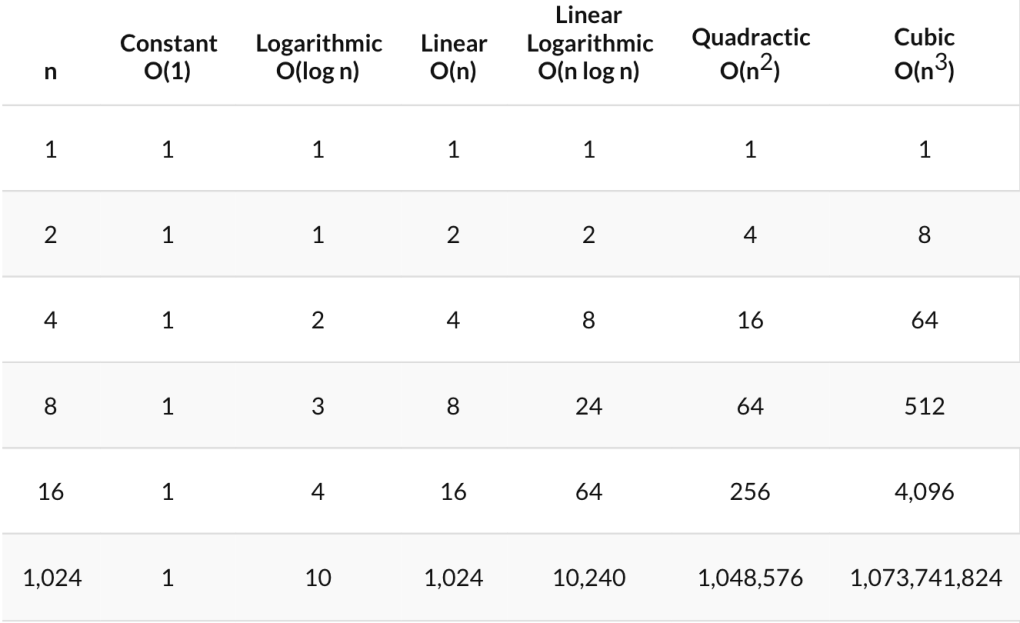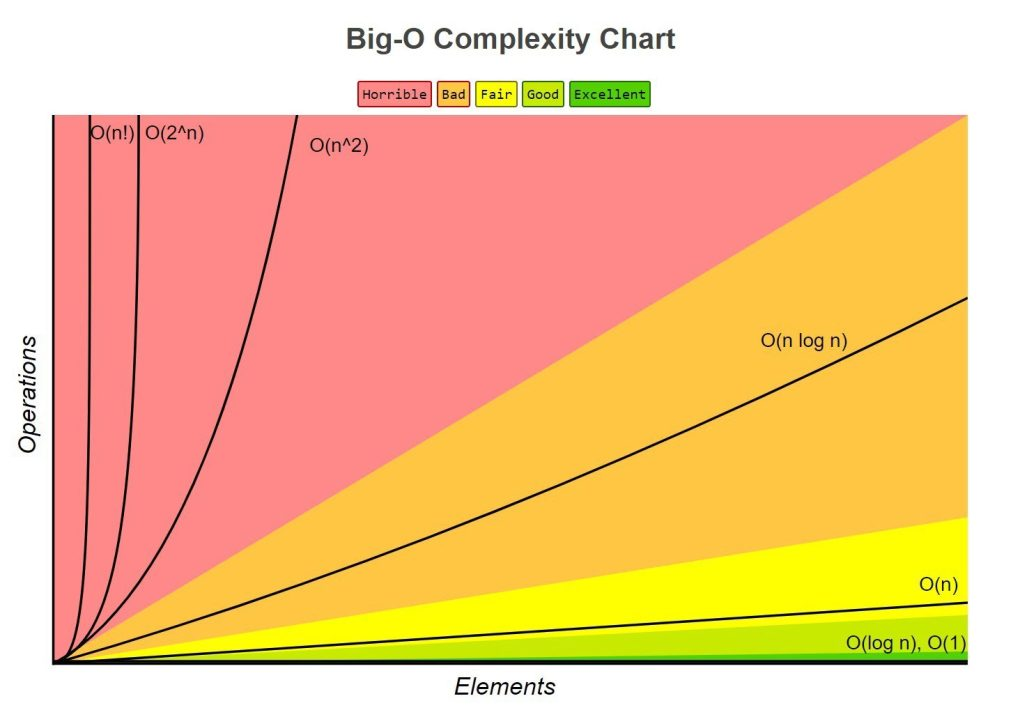# An overview of Big O notation

## Big O Notation

Big O notation describes the complexity of your code using algebraic terms. It is used to measure and compare the worst-case scenarios of algorithms theoretically. We use this notation to talk about how long an algorithm takes to run (time complexity) or how much memory an algorithm uses(space complexity). This notation can be used to express best, worst and average-case running time of an algorithm.

### Big Theta

Big Theta (Θ) represents an asymptotic tight bound. This happens when the upper bound(Big O) and lower bound(Big Omega or Ω ) are equal.

Example:

```for(int i=0; i<n; i++)
{
printf("%i", i);
}
```

The code above will always print the value of i n times so its upper bound or worst case is `O(n)`. The best case for this code is the same, i.e `Ω(n)`, so its runtime can be said to be `Θ(n)`.

### Big Omega

Big Omega (Ω) represents the best case scenario or the lower bound of an algorithm’s complexity. The lower bound of the loop below could be `Ω(1)` because if `some_condition` is true, the function won’t run `n` times. In other words, this function will run in constant time at best and `n` times at worst.

```for(int i = 0; i < n; i++)
{
if(some_condition)
{
break;
}
some_function(i);

}
```

## Worst case scenarios

The following are common worst case scenarios:

### O(1) -> Constant time

O(1) means that it takes a constant time to run an algorithm, regardless of the size of the input. A function that takes the same amount of time to process 10 items as well as a million items is said to run in O(1). Examples of O(1) algorithms:

• printing the first item in a list
• accessing array via an index
• accessing a value in a dictionary
```small_list = [1,2,3,4]
big_list = [1,2,3,4,...,100000000]

def find_first_index(n)
return n
```

The `find_first_index` will run in constant time(i.e it’ll go through the same number of steps) regardless of the size of the list used as its input.

### O(n) -> Linear Time

O(n) means that the run time increases proportionally to the size of input. An example of an O(n) operation is traversing through an array from start to finish. The number of operations it takes to loop through an array of length `n` is directly related to the size of `n`.

```int small_array = [1,2,3,4,5,6];
int big_array = [1,2,3,4,5,...1000];

void printValues(n)
{
for (int i = 0; i < sizeof(n); i++)
{
printf("%i", i);
}
}
```

Other examples of O(n) operations:

• linear search
• deleting of a specific element in an unsorted array
• comparing two strings

### O(log n) -> Logarithmic time

O(log n) means that the running time grows in proportion to the logarithm of the input size. The run time barely increases as you exponentially increase the input. Every time n doubles, the number of operations only increases by 1.

```small_num = 100
big_num = 1000

def count_operations(n):
operations = 0
i = 1

while i < n:
i = i * 2
operations += 1

return operations

print(count_operations(small_num),end="\n\n")
print(count_operations(big_num))
```

Another way to put this is time goes up linearly while the n goes up exponentially. Running the code above on inputs of 100 and 200 respectively returns 7 and 8 operations.

Some divide and conquer algorithms run in O( log n ) time. These include:

• binary search
• finding the smallest or largest value in a binary search tree

### O(n log n) -> Linearithmetic

Combination of linear and logarithmic complexity. Sorting algorithms that make use of the divide and conquer strategy fall into this category. These include:

• merge sort
• heapsort
• timsortThis chart shows that as the size of the input grows, the complexity or number of operations a function performs increases significantly. Our goal as developers is to keep this growth as low as possible.Graph showing how the number of operations increases with complexity.

O(n log n) falls between O(n^2) and O(n)

This represents a function whose complexity is directly proportional to the square of its input size. This type of complexity occurs when you perform nested iterations, i.e having a loop in a loop.
If you have an outer loop of n elements, the inner loop will run n times for each iteration of the outer loop which gives a total of n^2 runs. Can also be represented as O(n^2)

Examples:

• Bubble sort
• Insertion sort
• Selection sort

### O(2^n) Exponential Time

This occurs when the growth rate doubles with each addition to the input n. Any time n increases by 1, the number of operations executed doubles.

## Conclusion

Understanding Big O gives you insight on the best ways to solve problems and designing algorithms. I enjoyed learning these concepts and I hope this article will help you understand the concepts too.